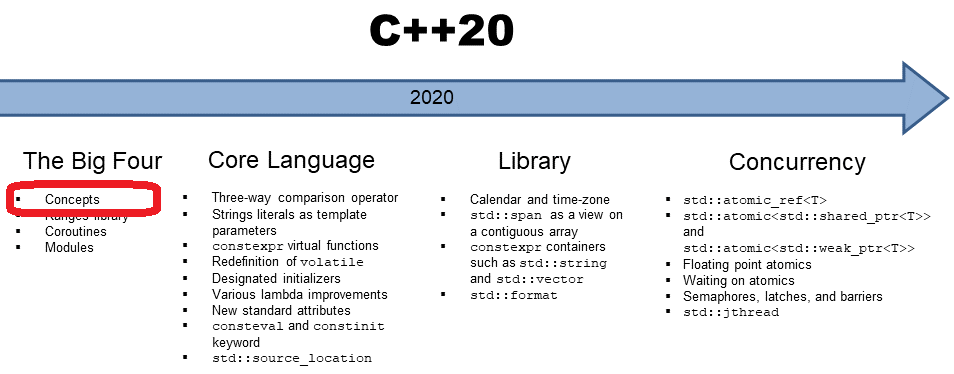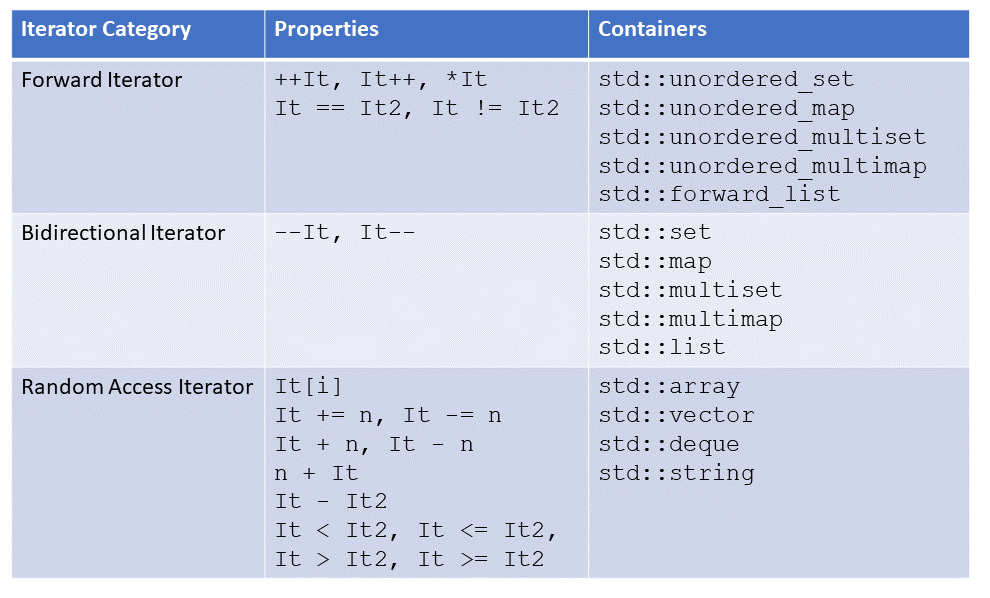# C++20: Concepts – Predefined Concepts

C++20 has many predefined concepts. Consequentially, before you define your concept, you should know the existing ones. This post gives you an overview of the predefined concepts. To get a general idea of concepts, read my previous postsDon’t reinvent the wheel. This golden rule also applies to concepts, by the way. The C++ Core Guidelines are very clear about this rule: T.11: Whenever possible use standard concepts

My information is from the newest C++20 draft: N4842. To find all predefined concepts is quite a job. Most concepts are in Chapter 18 (concepts library) and Chapter 24 (ranges library). Additionally, a few concepts are in Chapter 17 (language support library), chapter 20 (general utility library), chapter 23 (iterators library), and Chapter 26 (numerics library). The document also shows how the concepts are implemented.

Honestly, I was astonished that I found no concept of concurrency such as Lockable. Initially, the concepts were written in CamelCase or Wiki syntax. Now, they are written with underscores. E.g., DerivedFrom became derived_from.

Here are the concepts according to their ordering in the C++20 draft. I ignore this presentation the unique or auxiliary concepts. I also ignore the concepts from the ranges library. To understand them, you first have to understand the new ideas of the ranges library. I write about them when I write about the ranges library. The ranges library will follow the concepts immediately.

##Modernes C++ Mentoring

Be part of my mentoring programs:

• "Fundamentals for C++ Professionals" (open)
• "Design Patterns and Architectural Patterns with C++" (open)
• "C++20: Get the Details" (reopens December 2023)
• Do you want to stay informed about my mentoring programs: Subscribe via E-Mail.

I must warn you: if you don’t like technical posts, you probably don’t like this post.

## Language support library

This section has one interesting concept three_way_comparable to support the three-way comparison operator

If you want it more formal. Let a and b values of type T. They are essential three_way_comparable only if:

• (a <=> b == 0) == bool(a == b) is true,
• (a <=> b != 0) == bool(a != b) is true,
• ((a <=> b) <=> 0) and (0 <=> (b <=> a)) are equal,
• (a <=> b < 0) == bool(a < b) is true,
• (a <=> b > 0) == bool(a > b) is true,
• (a <=> b <= 0) == bool(a <= b) is true,
• (a <=> b >= 0) == bool(a >= b) is true, and

## Concepts Library

I assume these are the concepts we mainly use.

### Language-related concepts

This section has around 15 concepts that should be self-explanatory. These concepts express relationships between types, type classifications, and fundamental type properties. Their implementation is often directly based on the corresponding function from the type-traits library. I rearranged them and added a few words if necessary.

• same_as
• derived_from
• convertible_to
• common_reference_with: common_reference_with<T, U> must be well-formed, and T and U must be convertible to a reference type C
• common_with: similar to common_reference_with, but the common type C has not to be a reference type
• assignable_from
• swappable

#### Arithmetic

• integral
• signed_integral
• unsigned_integral
• floating_point

Their definition is straightforward:

```template<class T>
concept integral = is_integral_v<T>;

template<class T>
concept signed_integral = integral<T> && is_signed_v<T>;

template<class T>
concept unsigned_integral = integral<T> && !signed_integral<T>;

template<class T>
concept floating_point = is_floating_point_v<T>;```

• destructible
• constructible_from
• default_constructible
• move_constructible
• copy_constructible

### Comparison concepts

• boolean: specifies if a type T is usable as a truth value; interestingly, pointers, smart pointers, and types with an explicit conversion operator to bool are not boolean types.
• equality_comparable
• totally_ordered

Maybe you know it from your math. For values a, b, and c of type T, T models totally_ordered only if

• Exactly one of bool(a < b), bool(a > b), or bool(a == b) is true.
• If bool(a < b) and bool(b < c), then bool(a < c).
• bool(a > b) == bool(b < a).
• bool(a <= b) == !bool(b < a).
• bool(a >= b) == !bool(a < b).

### Object concepts

• movable
• copyable
• semiregular
• regular

Here is the definition of the four concepts:

```template<class T>
concept movable = is_object_v<T> && move_constructible<T> && assignable_from<T&, T> && swappable<T>;

template<class T>
concept copyable = copy_constructible<T> && movable<T> && assignable_from<T&, const T&>;

template<class T>
concept semiregular = copyable<T> && default_constructible<T>;

template<class T>
concept regular = semiregular<T> && equality_comparable<T>;
```

I have to add a few words. The concept movable requires for T that is_object_v<T> holds. This means, under the hood, that T is either a scalar, an array, a union, or a class.

I will implement the concept of semiregular and regular in future posts. Informally, a semiregular type behaves similarly to an int, and a regular type behaves similarly to an int and is comparable with ==.

### Callable concepts

• invocable
• regular_invocable: F models invocable and equality-preserving and does not modify the function arguments; equality-preserving means the F produces the same output when giving the same input
• predicate: F models a predicate if F models invocable and returns a boolean

## General Utility library

This chapter has only special memory concepts; therefore, I skip it.

## Iterators library

The iterators library has many important concepts. Here are the iterator categories:

• input_iterator
• output_iterator
• forward_iterator
• bidirectional_iterator
• random_access_iterator
• contiguous_iterator

The six categories of iterators correspond to the iterator concepts. The image shows the three most prominent iterator categories and the containers of the Standard Template Library, respectively.The following relation holds. A random-access iterator is a bidirectional iterator, and a bidirectional iterator is a forward iterator. A contiguous iterator is a random access iterator and requires that the elements of the container are stored contiguously in memory. This means std::array, std::vector, and std::string support contiguous iterators, but not std::deque.

### Algorithm concepts

• permutable: reordering of elements in place is possible
• mergeable: merging sorted sequences into an output sequence is possible
• sortable: permuting a sequence into an ordered sequence is possible

## Numeric library

The numeric library has the concept of a uniform_random_bit_generator. A  uniform_random_bit_generator g of type G has to return unsigned integers so that each value is equally probable. Additionally, the uniform random bit generator g of type G has to support the member functions G::min, and G::max.

What’s next?

With the next posts, it becomes more practical. I write about the definition of concepts such as integral, regular, and semiregular. Defining concepts is more than just putting constraints on type parameters.

Thanks a lot to my Patreon Supporters: Matt Braun, Roman Postanciuc, Tobias Zindl, G Prvulovic, Reinhold Dröge, Abernitzke, Frank Grimm, Sakib, Broeserl, António Pina, Sergey Agafyin, Андрей Бурмистров, Jake, GS, Lawton Shoemake, Jozo Leko, John Breland, Venkat Nandam, Jose Francisco, Douglas Tinkham, Kuchlong Kuchlong, Robert Blanch, Truels Wissneth, Kris Kafka, Mario Luoni, Friedrich Huber, lennonli, Pramod Tikare Muralidhara, Peter Ware, Daniel Hufschläger, Alessandro Pezzato, Bob Perry, Satish Vangipuram, Andi Ireland, Richard Ohnemus, Michael Dunsky, Leo Goodstadt, John Wiederhirn, Yacob Cohen-Arazi, Florian Tischler, Robin Furness, Michael Young, Holger Detering, Bernd Mühlhaus, Matthieu Bolt, Stephen Kelley, Kyle Dean, Tusar Palauri, Dmitry Farberov, Juan Dent, George Liao, Daniel Ceperley, Jon T Hess, Stephen Totten, Wolfgang Fütterer, Matthias Grün, Phillip Diekmann, Ben Atakora, Ann Shatoff, Rob North, Bhavith C Achar, and Marco Parri Empoli.

Thanks, in particular, to Jon Hess, Lakshman, Christian Wittenhorst, Sherhy Pyton, Dendi Suhubdy, Sudhakar Belagurusamy, Richard Sargeant, Rusty Fleming, John Nebel, Mipko, Alicja Kaminska, Slavko Radman, and David Poole.

 My special thanks to EmbarcaderoMy special thanks to PVS-StudioMy special thanks to Tipi.buildMy special thanks to Take Up Code## Seminars

I’m happy to give online seminars or face-to-face seminars worldwide. Please call me if you have any questions.

### Standard Seminars (English/German)

Here is a compilation of my standard seminars. These seminars are only meant to give you a first orientation.

• C++ – The Core Language
• C++ – The Standard Library
• C++ – Compact
• C++11 and C++14
• Concurrency with Modern C++
• Design Pattern and Architectural Pattern with C++
• Embedded Programming with Modern C++
• Generic Programming (Templates) with C++

#### New

• Clean Code with Modern C++
• C++20

### Contact Me

Modernes C++ Mentoring,Tags:
0 replies